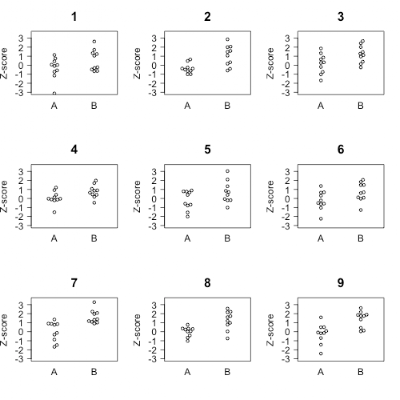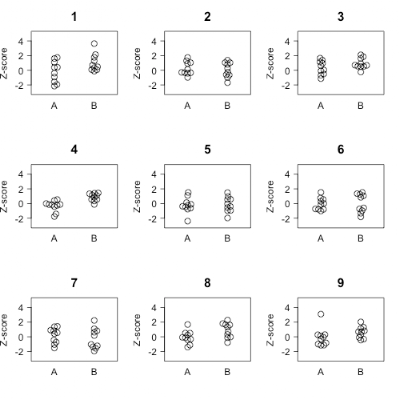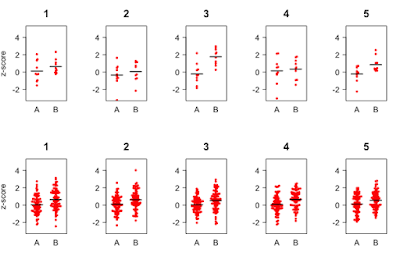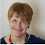## Thursday 21 December 2017

### Intuitive explanations of statistical concepts for novices #3

I'll be focusing here on the kinds of stats needed if you conduct an intervention study. Suppose we measured the number of words children could define on a 20-word vocabulary task. Words were selected so that at the start of training, none of the children knew any of them. At the end of 3 months of training, every child in the vocabulary training group (B) knew four words, whereas those in a control group (A) knew three words. If we had 10 children per group, the plot of final scores would look like Figure 1 panel 1.Figure 1. Fictional data to demonstrate concept of random error (noise)

In practice, intervention data never look like this. There is always unexplained variation in intervention outcomes, and real results look more like panel 2 or panel 3. That is, in each group, some children learn more than average and some less than average. Such fluctuations can reflect numerous sources of uncontrolled variation: for instance, random error will be large if we use unreliable measures, or there may be individual differences in responsiveness to intervention in the people included in the study, as well as things that can fluctuate from day to day or even moment to moment, such as people's mood, health, tiredness and so on.

The task for the researcher is to detect a signal – the effect of intervention – from noise – the random fluctuations. It is important to carefully select our measures and our participants to minimise noise, but we will never eliminate it entirely.

There are two key concepts behind all the statistics we do: (a) data will contain random noise, and (b) when we do a study we are sampling from a larger population. We can make these ideas more concrete through simulation.

The first step is to generate a large quantity of random numbers. Random numbers can be easily generated using the free software package R: if you have this installed, you can follow this demo by typing in the commands shown in italic at your console. R has a command, rnorm, that generates normally distributed random numbers. For instance:

rnorm(10,0,1)

will generate 10 z-scores, i.e. random numbers with mean of 0 and standard deviation of 1.You get new random numbers each time you submit the command, (unless you explicitly set something known as the random number seed to be the same each time). Now let's use R to generate 100,000 random numbers, and plot the output in a histogram. Figure 2 can be generated with the commands:

myz = rnorm(100000,0,1)
hist(myz)Figure 2: Distribution of z-scores simulated with rnorm

This shows that numbers close to zero are most common, and the further we get from zero in either direction, the lower the frequency of the number. The bell-shaped curve is a normal distribution, which we get because we opted to generate random numbers following a normal distribution using rnorm. (Other distributions of random number are also possible; you can see some options here).

So you might be wondering what we do with this list of numbers. Well, we can simulate experimental data based on this population of numbers by specifying two things:
1. The sample size
2. The effect size – i.e., Cohen's d, the mean difference between groups in standard deviation (SD) units.

Suppose we want two groups, A and B, each with a sample size of 10, where group B has scores that are on average 1 SD larger than group A. First we select 20 values at random from myz:

mydata = sample(myz, 20)

Next we create a variable corresponding to group, which is created by just making a variable, mygroup, that combines ten repeats of 'A' with ten repeats of 'B'.

mygroup = c(rep('A', 10), rep('B', 10))

Next we add the effect size, 1, to the last 10 numbers, i.e. those for group B

mydata[11:20] = mydata[11:20] + 1

Now we can plot the individual points clustered by group. First install and activate the beeswarm package to make a nice plot format:

install.packages('beeswarm')
library(beeswarm)

Then you can make the plot with the command:

beeswarm(mydata ~ mygroup)

The resulting plot will look something like one of the graphs in Figure 3. It won't be exactly the same as any of them because your random sample will be different from the ones we have generated. In fact, this is one point of this exercise: to show you how numbers will vary from one occasion to another when you sample from a population.

If you just repeatedly run these lines, you will see how things vary just by chance:

mydata = sample(myz, 20)
mydata[11:20] = mydata[11:20] + 1
beeswarm(mydata ~ mygroup)Figure 3: Nine runs of simulated data from 2 groups: A comes from population with mean score of 0 and B from population with mean score of 1
Note how in Figure 3, the difference between groups A and B is far more marked in runs 7 and 9 than in runs 4 and 6, even though each dataset was generated by the same script. This is what is meant by the 'play of chance' affecting experimental data.

Now let's look at Figure 4, which gives output from another nine runs of a simulation. This time, some runs were set so that there was a true effect of intervention (by adding .6 to values for group B) and some were set with no difference between groups. Can you tell which simulations were based on a real effect?Figure 4: Some of these runs were generated with effect size of .6, others had no difference between A and B
The answer is that runs 1, 2, 4, 8 and 9 came from runs where there was a real effect of .6 (which, by the standard of most intervention studies is a large effect). You may have identified some of these runs correctly, but you may also to have falsely selected run 3 as showing an effect. This would be a false positive, where we wrongly conclude there is an intervention effect when the apparent superiority of the intervention group is just down to chance. This type of error is known as a type I error. Run 2 looks like a false negative – we are likely to conclude there is no effect of intervention, when in fact there was one. This is a type II error. One way to remember this distinction is that a type I error is when you think you've won (1) but you haven't.

The importance of sample size
Figures 3 and 4 demonstrate that, when inspecting data from intervention trials, you can't just rely on the data in front of your eyes. Sometimes, they will suggest a real effect when the data are really random (type I error) and sometimes they will fail to reveal a difference when the intervention is really effective (type II error). These anomalies arise because data incorporates random noise which can generate spurious effects or mask real effects. This masking is particularly problematic when samples are small.

Figure 5 shows two sets of data: the top panel and the bottom panel were derived by the same simulation, the only difference being the sample size: 10 per group in the top panels, and 80 per group in the bottom panels. In both cases, the simulation specified that group B scores were drawn from a population that had higher scores than group A, with an effect size of 0.6.  The bold line shows the group average. The figure shows that the larger the sample, the closer the results from the sample will agree with the population from which it was drawn.Figure 5: Five runs of simulation where true effect size = .6

When samples are small, estimates of the means will jump around much more than when samples are large. Note, in particular, that with the small sample size, on the third run, the mean difference between A and B is overestimated by about 50%, whereas in the fourth run, the mean for B is very close to that for A.

In the population from which these samples are taken the mean difference between A and B is 0.6, but if we just take a sample from this population, by chance we may select atypical cases, and these will have a much larger impact on the observed mean when the sample is small.

In my next post, I will show how we can build on these basic simulations to get an intuitive understanding of p-values.

P.S. Scripts for generating the figures in this post can be found here.

1.2.